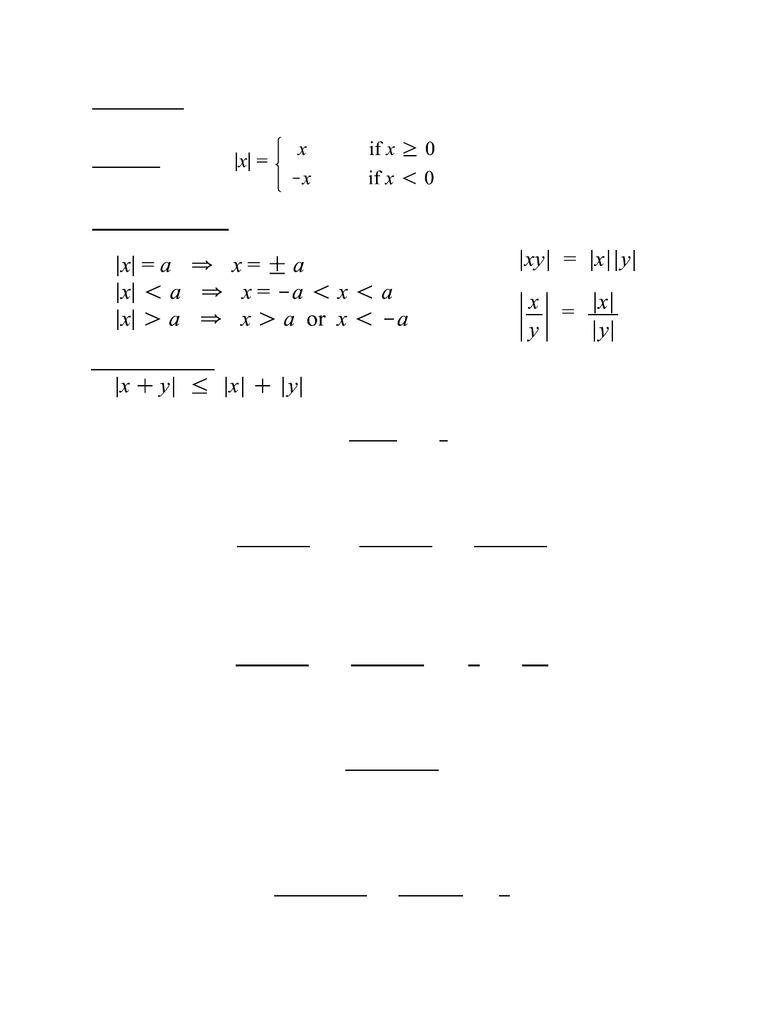# MATH137 Study Guide - Midterm Guide: Oliver Heaviside, Triangle Inequality, Entire Function

138 views21 pages
School
Department
Course
ProfessorWaterloo SOS
Fall 2010
MATH 137 Midterm Review
October 23rd 2010
Aaron
Unlock document

This preview shows pages 1-3 of the document.
Unlock all 21 pages and 3 million more documents.FUNCTIONS AND ABSOLUTE VALUE
REVIEW OF FUNCTIONS
A function f, assigns exactly one value to every element x. We can think of x as the function’s
input and y as its output. For our purposes, we can use y and f(x) interchangeably. In Calculus 1,
we deal with functions taking elements of the real numbers as inputs and outputting real
numbers. We are primarily concerned with using graphs as visual representations of functions.
Here are some definitions that are useful to keep in mind:
Domain: The set of elements x that can be inputs for a function f
Range: The set of elements y that are outputs of a function f
Increasing Function: A function is increasing over an interval A if for all , the property
 holds.
Decreasing Function: A function is decreasing over an interval A if for all , the property
 holds.
Even Function: A function with the property that for all values of x: 
Odd Function: A function with the property that for all values of x: 
A function is neither even nor odd if it does not satisfy either of these properties. When
sketching, it is helpful to keep in mind that even functions are symmetric about the y-axis and
that odd functions are symmetric about the origin (0, 0).
Unlock document

This preview shows pages 1-3 of the document.
Unlock all 21 pages and 3 million more documents.ABSOLUTE VALUE
Definition:
Properties and Rules:
Example. Given that show that 

First, we split the fraction and apply the triangle inequality to obtain:




Note that  for any value of x. Therefore, if we replace the denominator with 5, we
are shrinking it and thereby making the entire rational expression larger. Hence:



After applying properties of absolute value, we can obtain the following expression:

By applying the definition of absolute value, we get that |-1| = 1 and |5| = 5. Since |x| < 2, as
provided in the question, we can safely substitute 2 for x in the expression. Thus, we obtain:



Triangle Inequality:
Unlock document

This preview shows pages 1-3 of the document.
Unlock all 21 pages and 3 million more documents.